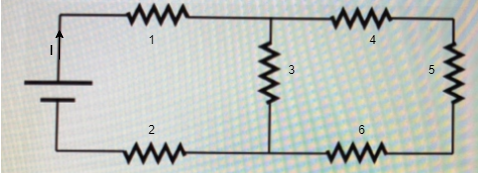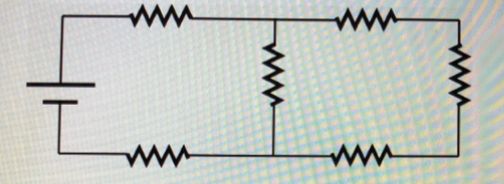# Problem: Identify the maximum number of unique currents possible for the circuit shown in the figure. A. two B. three C. four D. six E. seven

###### FREE Expert Solution

Let's take all the resistances to be equal.

We label the resistors as:95% (398 ratings)###### Problem Details

Identify the maximum number of unique currents possible for the circuit shown in the figure.A. two

B. three

C. four

D. six

E. seven

Frequently Asked Questions

What scientific concept do you need to know in order to solve this problem?

Our tutors have indicated that to solve this problem you will need to apply the Intro to Current concept. You can view video lessons to learn Intro to Current. Or if you need more Intro to Current practice, you can also practice Intro to Current practice problems.